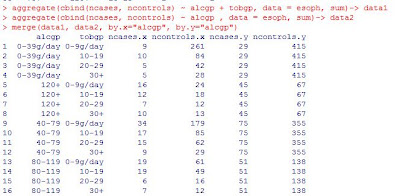## Wednesday, July 18, 2012

### HAVING and CALCULATED in PROC SQL

HAVING: The distinction between HAVING and the WHERE clause is that HAVING conditions can reference summary statistics and are evaluated after aggregations are performed. Thus they take effect “downstream,” on the output side of the process. So we can use WHERE statement in the data sets to replace this HAVING, as is done below.

CALCULATED: if you want to use the calculated result during the SQL select clause, you need to add CALCULATED otherwise there will be error like: ERROR: The following columns were not found in the contributing tables: price_range

In the following the first one is faster than the second, although they generate the same results.

``proc sql;       create table outdata.event_trends1 as       select event_id, active_max_price-active_min_price as price_range, max(calculated price_range) as max_range, datepart(trend_time) as trend_date       from fansnapd.event_trends       group by event_id       having calculated price_range=max_range       order by event_id;quit;proc sql;       create table outdata.event_trends(where=(price_range=max_range)) as       select event_id, active_max_price-active_min_price as price_range, max(calculated price_range) as max_range, datepart(trend_time) as trend_date       from fansnapd.event_trends       group by event_id       order by event_id;quit;``

### Variables order in the KEEP RENAME WHERE INDEX clause in the data sets

Variables order in the KEEP RENAME WHERE INDEX clause in the data sets:

1) KEEP = variables name exactly the same as original data set
2) RENAME change the original variable name to the new one
3) WHERE INDEX should use the new name

In the following clicks table has variable event_id guid is_cpa.

proc sort data= clicks (keep=event_id guid is_cpa rename=(event_id=id is_cpa=cpa guid=fsid)) where=(cpa=1) out=test1(index=(fsid));
by id;
run;

## Thursday, July 12, 2012

### SAS study notes: index in sas: when to use index, how to create index, how to modify index / datasets / variables without losing index

Index

When to use index:

1: yield faster access to small subsets of obs for WHERE processing

2: return obs in sorted order for BY processing

3: perform table lookup operations

4: join obs

5: modify obs

Simple index: (index=(myindex)) or (index=(lastname firstname))

Composite index: (index=(comp_index=(lastname firstname)))

Pcoc datasets library=libref;

Modify sas_data_set;

Index delete index_name;

Index create index_specification;

Quit;

Proc sql;

Create index index_name on table_name;

Drop index index_name from table_name;

Quit;

 Task Effect Add observation(s) to data set Value/identifier pairs are added to index(es). Delete observation(s) from data set Value/identifier pairs are deleted from index(es). Update observation(s) in data set Value/identifier pairs are updated in index(es). Delete data set The index file is deleted. Rebuild data set with DATA step The index file is deleted. Sort the data in place with the FORCE option in PROC SORT The index file is deleted.

Use PROC DATASETS to copy, rename(change) datasets, rename variables

DATA SAS-data-file-name (INDEX=

(index-specification-1</UNIQUE><...index-specification-n</UNIQUE>>));

SET SAS-data-set-name ;

RUN;

PROC DATASETS LIBRARY=libref ;

MODIFY SAS-data-set-name;

INDEX DELETE index-name ;

INDEX CREATE index-specification;

QUIT;

PROC SQL;

CREATE INDEX index-name

ON table-name(column-name-1<...,column-name-n>);

DROP INDEX index-name FROM table-name ;

QUIT;

PROC CONTENTS DATA=SAS-data-set-name;

RUN;

PROC DATASETS <LIBRARY=libref> ;

CONTENTS DATA=SAS-data-set-name;

QUIT;

PROC DATASETS LIBRARY=old-libref ;

COPY OUT=new-libref;

SELECT SAS-data-set-name;

QUIT;

PROC COPY OUT=new-libref IN=old-libref ;

SELECT SAS-data-set-name(s);

RUN;

QUIT;

PROC DATASETS LIBRARY=libref ;

CHANGE old-data-set-name = new-data-set-name;

QUIT;

PROC DATASETS LIBRARY=libref ;

MODIFY SAS-data-set-name;

RENAME old-var-name-1 = new-var-name-1 ;

<...old-var-name-n = new-var-name-n> ;

QUIT;

## Thursday, July 5, 2012

### R vs SAS 1: R aggregate v.s. SAS proc summary

In SAS, it's convenient to calculate mean/sum alike statistics over different subset of the original data using proc summary.

In R we can get the similar result using function "aggregate", or use "tapply" for simple condition.

Example:

``library(stats)aggregate(cbind(ncases, ncontrols) ~ alcgp + tobgp, data = esoph, sum)-> data1aggregate(cbind(ncases, ncontrols) ~ alcgp , data = esoph, sum)-> data2merge(data1, data2, by.x="alcgp", by.y="alcgp")``

gives us:We can get this from SAS:
``data a;     infile "./esoph.txt" firstobs=2;     input agegp \$ alcgp \$ tobgp \$ ncases ncontrols;run;proc print data=a;run;proc summary data=a ;     class alcgp tobgp;     var ncases ncontrols;     output out=temp(drop=_freq_) sum=;run;proc print data=temp;run;proc sort data=temp;     by alcgp;run;data final(drop=_type_);     merge temp(where=(_type_=3)) temp(where=(_type_=2) rename=(ncases=tot_ncases ncontrols=tot_ncontrols));     by alcgp;run;proc print data=final;run;``

The output is: